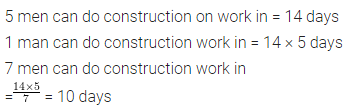ML Aggarwal Class 6 Solutions Chapter 8 Ratio and Proportion Objective Type Questions for ICSE Understanding Mathematics acts as the best resource during your learning and helps you score well in your exams.

## ML Aggarwal Class 6 Solutions for ICSE Maths Chapter 8 Ratio and Proportion Objective Type Questions

Mental Maths
Question 1.
Fill in the blanks:
(i) In the ratio 3 : 5, the first term is …………. and second term is ………..
(ii) In a ratio, the first term is also called ……….. and second term is also called …….
(iii) If two terms of a ratio have no common factor (except 1), then the ratio is said to be in …….
(iv) To simplify a ratio, we divide the two terms by their …….
(v) The simplest form of the ratio 8 : 12 is ……
(vi) 90 cm : 1.5 m = ……….
(vii) Method of comparison of two quantities of the same kind (in same units) by division is known as …………
(viii) When two ratios are equal, they are said to be in ………
(ix) When four quantities are in proportion, then the product of ………… is equal to product of middle terms.
(x) 4.5 omo is equal to ………
Solution:Question 2.
State whether the following statemtns are true (T) or false (F):
(i) Ratio exists only between two quantities of the same kind.
(ii) Ratio has no units.
(iii) If a ≠ b, then the ratio a: bis different from the ratio b : a.
(iv) If we multiply or divide both terms of a ratio by the same non-zero number, then the ratio remains the same.
(v) The ratio a: b is said to be in simplest form if HCF of a and b is 1.
(vi) In some situations, comparison of two quantities (of same kind) by difference does not make much sense.
Solution: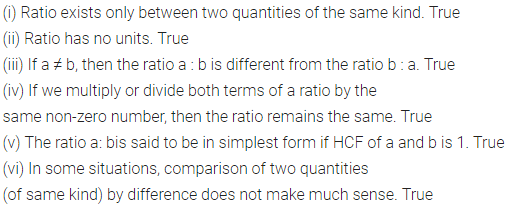Multiple Choice Questions
Choose the correct answer from the given four options (3 to 18):
Question 3.
A ratio equivalent to 5 : 7 is
(a) 10:21
(b) 15 : 14
(c) 20 : 28
(d) 25 : 49
Solution: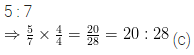Question 4.
The ratio 384 : 480 in the simplest form is
(a) 2 : 5
(b) 3 : 5
(c) 5 : 4
(d) 4 : 5
Solution:Question 5.
The ratio of 20 minutes to 1 hour is
(a) 20 : 1
(b) 1 : 3
(c) 1 : 4
(d) 2 : 5
Solution: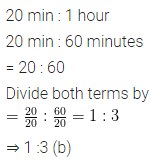Question 6.
The ratio of 150 g to 2 kg is
(a) 75 : 1
(b) 40 : 3
(c) 3 : 40
(d) 3 : 200
Solution: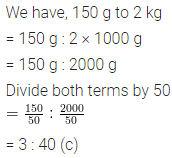Question 7.
In a class of 40 students, 25 students play cricket and the remaining play tennis. The ratio of number of students playing crickets to the number of students playing tennis is
(a) 5 : 8
(b) 5 : 3
(c) 3 : 5
(d) 8 : 5
Solution: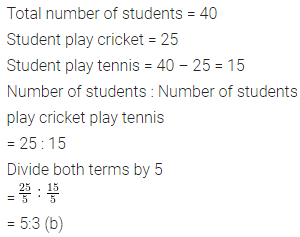Question 8.
Two numbers are in the ratio 3 : 5. If the sum of numbers is 144, then the smaller number is
(a) 54
(b) 72
(c) 90
(d) 48
Solution: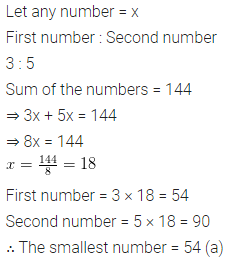Question 9.
The ratio of number of girls to the number of boys in a class is 5 : 4. If there are 25 girls in the class, then the number of boys in the class is
(a) 15
(b) 20
(c) 30
(d) 40
Solution:Question 10.
The ratio of the number of sides of a square and the number of edges of a cube is
(a) 1 : 2
(b) 1 : 3
(c) 1 : 4
(d) 2 : 3
Solution: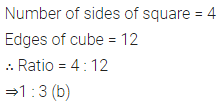Question 11.
In shelf, the books with green cover and that with brown cover are in the ratio 2:3. If there are 18 books with green cover, then the number of books with brown cover is
(a) 12
(b) 24
(c) 27
(d) 36
Solution: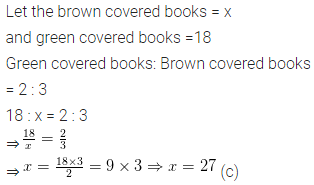Question 12.
In a box, the ratio of the number of red marbles to that of blue marbles is 4 :7. Which of the following could be the total number of marbles in the box?
(a) 14
(b) 21
(c) 22
(d) 28
Solution:Question 13.
If a, b, c and d are in proportion, then
(a) ab = cd
(b) ad = be
(c) ac = bd
(d) none of these
Solution: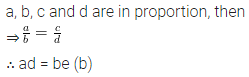Question 14.
If the weight of 5 bags of rice is 272 kg, then the weight of 1 bag of rice is
(a) 50.4 kg
(b) 54.4 kg
(c) 54.004 kg
(d) 54.04 kg
Solution: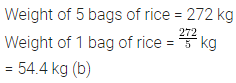Question 15.
If 7 pencils cost ₹35, then the cost of one dozen pencils is
(a) ₹60
(b) ₹70
(c) ₹30
(d) ₹5
Solution: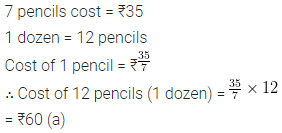Question 16.
The ratio 2 : 3 expressed as percentage is
(a) 40%
(b) 60%
(c) $$66 \frac{2}{3} \%$$
(d) $$33 \frac{1}{3} \%$$
Solution: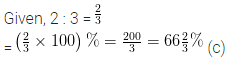Question 17.
0.025 when expressed as percentage is
(a) 250%
(b) 25%
(c) 4%
(d) 2.5%
Solution: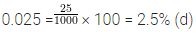Question 18.
In a class, 45% of the students are girls. If there are 18 girls in the class, then the total number of students in the class is
(a) 44
(b) 40
(c) 36
(d) 30
Solution: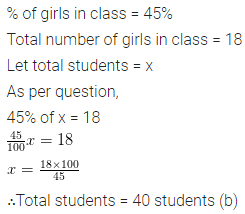Value Based Questions
Question 1.
Students of a colony decided to go to an old age home in their vicinity to wish Happy New year and get blessings from old people.
They carried the following items with them:
Bouquets 63, New Year Cards 70 and chocolates bars 140. Answer the following questions:
(i) What is the ratio of number of bouquets to the number of chocolate bars?
(ii) What is the ratio of number of cards to the number of sum of all items?
Solution: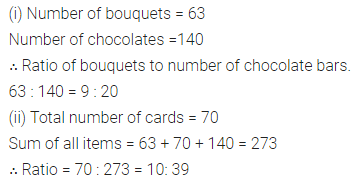Higher Order Thinking Skills (Hots)
Question 1.
Divide ₹6000 among Irfan, Nagma and Ishan in the raito 3 : 5 : 7.
Solution: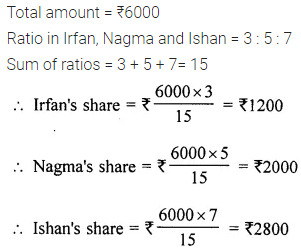Question 2.
Sapna weighs 54 kg on earth and 9 kg on moon. If a monkey weighs 3.5 kg on moon, then how much will it weigh on the earth?
Solution: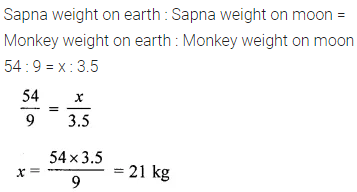Question 3.
If 5 men can do a certain construction work in 14 days, then how long will 7 men take to complete the same construction work?
Solution: